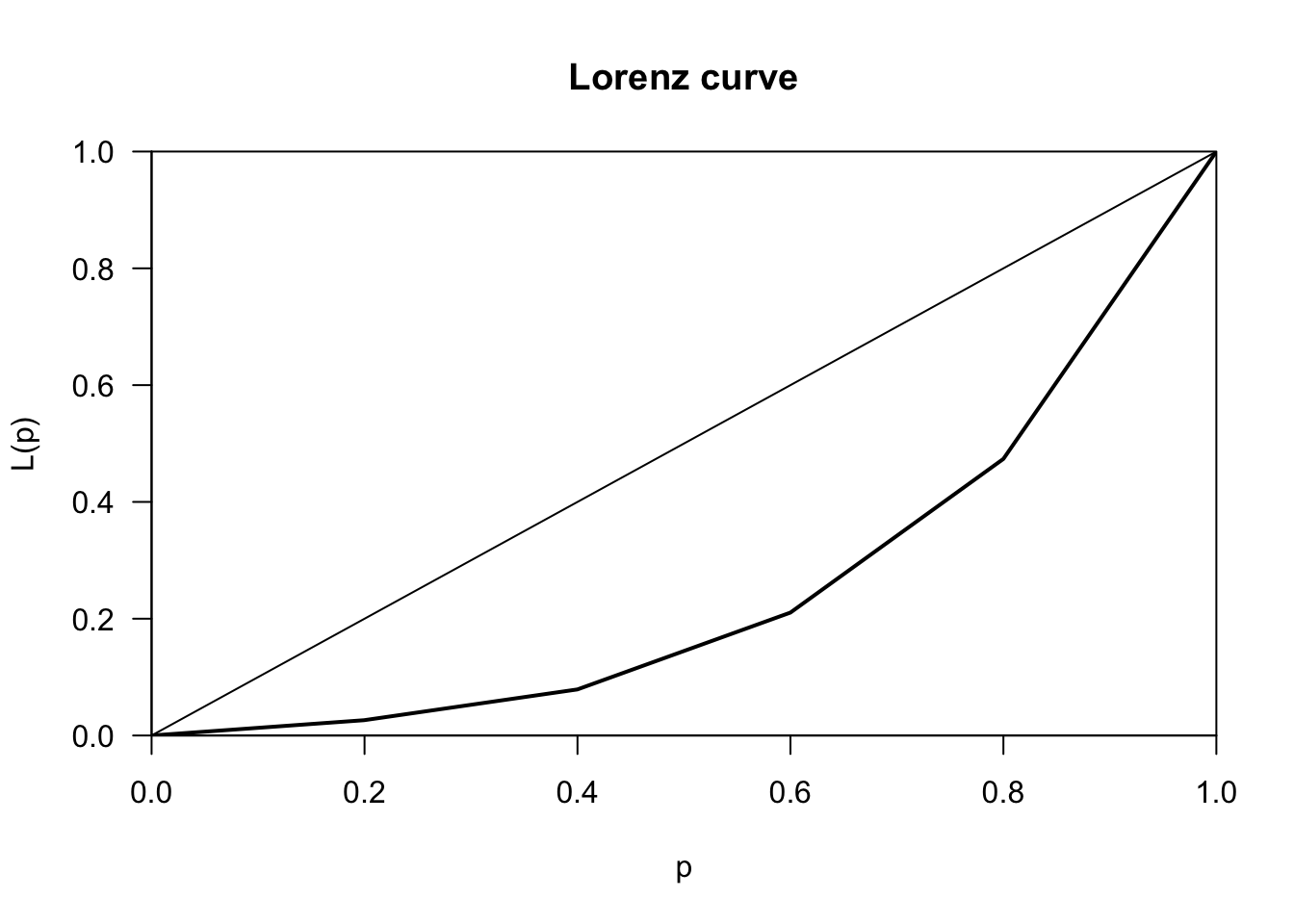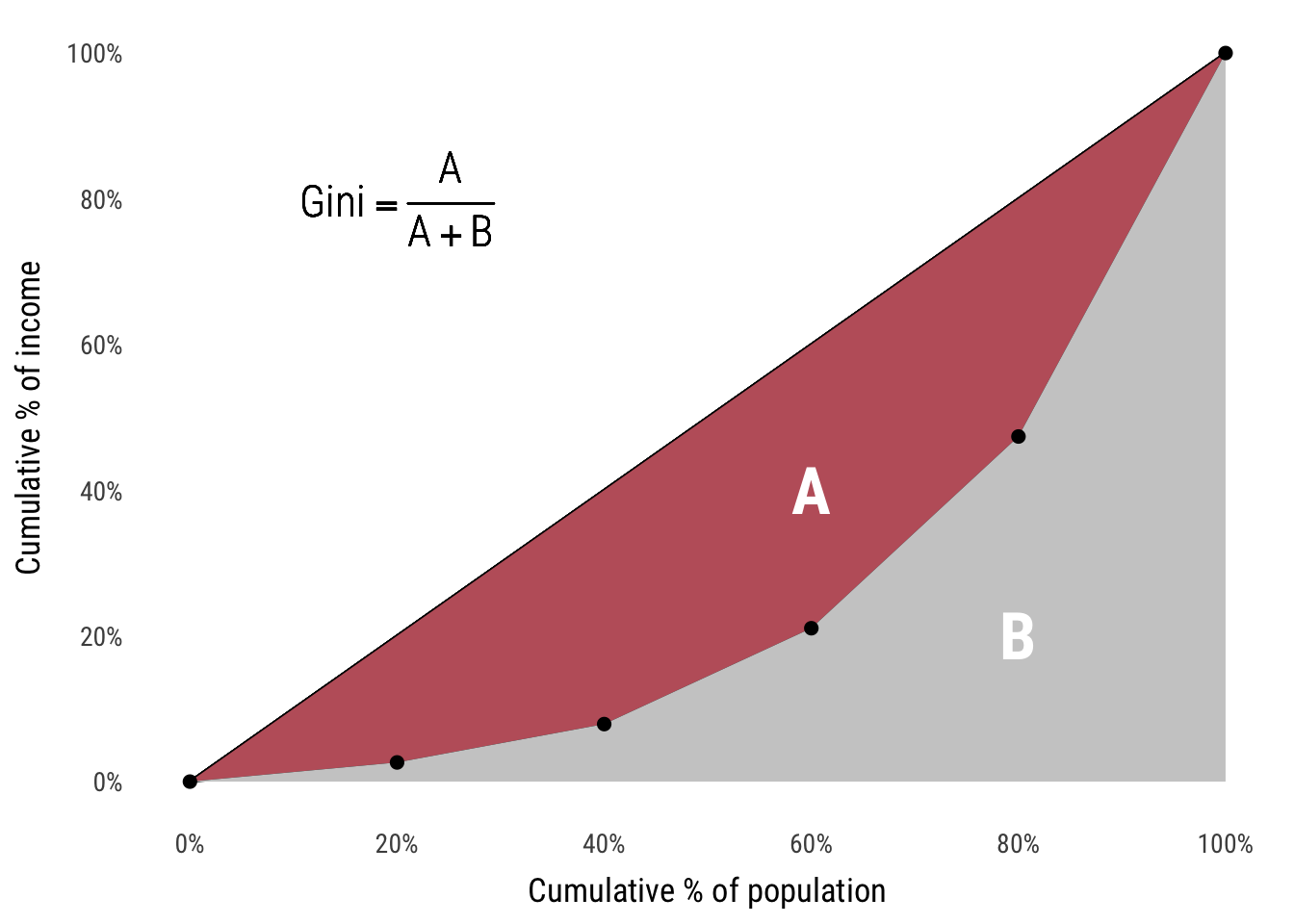# Markets and Measurement II

Materials for class on Wednesday, January 10, 2018

## Slides

### Indicators

$\text{Real GDP} = \frac{\text{Nominal GDP}}{\text{Price Index / 100}}$

## Measuring inequality with Gini coefficients

Calculating Gini coefficients with R is trivial with the ineq package—create a vector of incomes and feed it into ineq(). Here’s what you do for a fictional country with the following incomes:

Person Income
1 $10,000 2$20,000
3 $50,000 4$100,000
5 $200,000 library(ineq) incomes <- c(10000, 20000, 50000, 100000, 200000) ineq(incomes, type = "Gini") ##  0.4842105 There are other types of inequality measures too. The default is Gini, but if you look at the documentation of ineq() with ?ineq, you can see the others. You can plot the Lorenz curve easily too: plot(Lc(incomes))Alternatively you can extract the Lorenz curve that’s returned from Lc() and use ggplot2 to create a nicer plot: library(tidyverse) # This file is on GitHub at https://github.com/andrewheiss/econw18.classes.andrewheiss.com/blob/master/lib/graphics.R source(file.path(here::here(), "lib", "graphics.R")) # Load theme and colors lorenz <- Lc(incomes) plot_data <- data_frame(prop_population = lorenz$p,
prop_income = lorenz\$L)

plot_labels <- tribble(
~x, ~y, ~label,
0.6, 0.4, "A",
0.8, 0.2, "B"
)

ggplot(plot_data, aes(x = prop_population, y = prop_income)) +
geom_line() +
geom_segment(x = 0, xend = 1, y = 0, yend = 1) +
geom_ribbon(aes(ymax = prop_population, ymin = prop_income), fill = nord_red) +
geom_ribbon(aes(ymax = prop_income, ymin = 0), fill = "grey80") +
geom_point(size = 2) +
geom_text(x = 0.2, y = 0.8, label = "Gini == frac(A, A + B)", parse = TRUE,
size = 6, family = "Roboto Condensed Light") +
geom_text(data = plot_labels, aes(x = x, y = y, label = label),
color = "white", size = 9, family = "Roboto Condensed Bold") +
labs(x = "Cumulative % of population", y = "Cumulative % of income") +
scale_x_continuous(labels = scales::percent, breaks = seq(0, 1, 0.2)) +
scale_y_continuous(labels = scales::percent, breaks = seq(0, 1, 0.2)) +
theme_econ(13) +
theme(panel.grid = element_blank())